Next: Heat Equation, General Case Up: HEAT EQUATION (TRANSIENT CONDUCTION) Previous: HEAT EQUATION (TRANSIENT CONDUCTION)

## Heat Equation, 1-D Rectangular Case.

Consider the following boundary-value problem for temperature in a 1-D body in rectangular coordinates: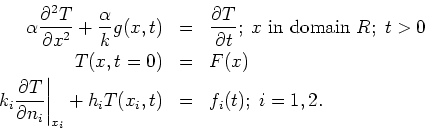Note that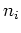is the outward normal on boundary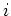. The convective (type 3) boundary conditions are specified on boundaries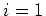and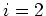. Boundary conditions of type 1 or 2 are also included by this relationship by taking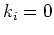or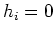, respectively, on boundariesor.

The Green's Function Solution Equation for temperature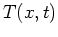is given by: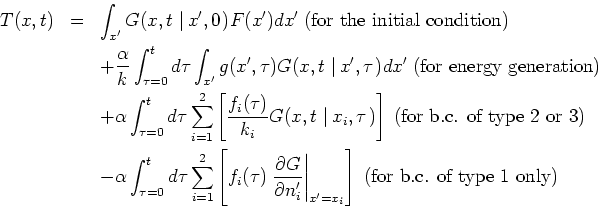The spatial integrals should be evaluated over the whole body, for example, on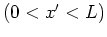for a plate, or over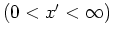for a semi-infinte body. The sumations in the boundary condition terms represent at most two boundaries.

The same GF appears in each integral term, evaluated at the source location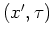appropriate for that integral term. For example, in the initial-condition integral the GF is evaluated at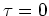; in a boundary-condition integral the GF is evaluated at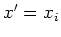,

2004-01-31